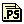#### by Tae-Kyung Hong, PhD Thesis, The Australian National University, (2003)[full text, PDF](36446k)|[full text, Gzipped PS](9511k)

A wavelet-based method is developed for the numerical modelling of acoustic and elastic wave propagation. Several techniques are implemented together to develop the method. Using a displacement-velocity formulation and treating spatial derivatives with linear operators based on wavelet transform, the wave equations are rewritten as a system of equations whose evolution in time is controlled by first-order derivatives. The linear operators for the spatial derivatives are treated in wavelet bases by projection with a wavelet transform. The discretized solution in time can then be represented in an explicit recursive form using a semigroup approach. Absorbing boundary conditions are considered implicitly by including attenuation terms in the governing equations, and the traction-free boundary conditions can be implemented by augmenting the system of equations with equivalent force terms at the boundaries.

This wavelet-based method is applied to acoustic, SH and P-SV waves for several 2-D models with rigid or traction-free boundary conditions, and numerical results are compared with the analytic solutions. Also, the wavelet-based method is extended to problems with topography using a grid-mapping technique. The method is stable even in the applications to highly-varying topography problems and generates accurate responses. The wavelet-based approach is also appropriate for modelling in complex media with highly perturbed random media or with strong heterogeneities. The new technique is shown to be suitable for accurate and stable modelling of wave propagation in general complex media.

The wavelet approach is then expanded to models with localised heterogeneity, with significant contrasts with their surroundings. We consider zones with both lowered wavespeed such as a fault gouge zone and elevated wavespeeds as in a subduction zone. In each of these situations the source lies within the heterogeneity. The representation of the source has therefore been adapted to work directly in a heterogeneous environment, rather than using a locally homogeneous zone around the source. This extension also allows the wavelet method to be used with a wider variety of sources, e.g., propagating sources. For the fault zone we consider both point and propagating sources through a moment tensor representation, and reveal significant trapped waves along the gouge zone as well as permanent displacements. For subduction zones a variety of effects are produced depending on the depth and position of the source relative to the subducting slab. A variety of secondary waves, such as reflected and interface waves, can be produced in wavetrains at regional distances and tend to be more important for greater source depth.

The high stability and accuracy of the wavelet-based method in highly perturbed media allows this approach to be exploited for the investigation of seismic-wave scattering in several stochastic (Gaussian, exponential, von Karman) random media. The scattering attenuation depends on the correlation distance of the random heterogeneities, the velocity ratio of P and S waves, and the frequency content of the incident waves. Theoretical attenuation variation is derived for the comparisons with numerical results by using the first-order Born approximation. The minimum scattering angle for these stochastic media is found to be in the range 60-90 degrees, and it appears that methods such as finite-differences may overestimate scattering attenuation when the level of the heterogeneity is high.

The characteristics of scattering attenuation patterns in elastic waves are investigated using the theoretical expressions for P and S waves. S waves lose more energy in the low frequency range (fa<=1 km/s, where fa is the normalized frequency) than P waves, and the phenomenon is reversed at high frequency range. The frequency dependency of seismic scattering makes the scattering attenuation ratio increase with frequency at 0.1<fa<2 km/s, and the ratio of P and S scattering is nearly constant outside these ranges of normalized frequency. The minimum ratio is determined to be about 0.4 and the maximum ratio increases with the Hurst number (nu) for von Karman random media (including exponential random media) from 1 (nu=0.05) to 1.7 (nu=0.5). Gaussian random media display a steep change in the P/S scattering ratio and may not be suitable for the representation of natural random heterogeneities in the earth. With an appropriate choice of the Hurst number, the von Karman model can reproduce the random heterogeneities of the crust.

From complementary studies on scatterings of acoustic and SH waves in stochastic random media, the effects of physical-parameter perturbation on scattering are resolved. Due to the difference in the form of the equations between acoustic and elastic waves, i.e., the differences in the placement of the density and Lame coefficients, there are characteristic differences in scattering patterns and the attenuation rates. The perturbation in the density in elastic waves introduces additional energy loss in primary waves, and the energy loss is proportional to the magnitude of the density perturbation.

Finally, elastic waves are modelled in media with randomly distributed fluid-filled circular cavities, which is a challenging problem for numerical techniques. The energy dissipation of the primary waves is proportional to the scale of the cavities. Theoretical attenuation variations for media with circular cavities, which may be filled with any materials (e.g., vacuum, fluid, elastic materials), have been formulated and they are compared with the numerical results for the media with fluid-filled cavities. The numerically estimated attenuation rates agree well with the theoretical variation. The attenuation rates increase linearly with normalized wavenumber, unlike those in stochastic random media that display a parabolic trend for the normalized wavenumber. Also, the normalized attenuation rates are identical between those measured from media with a same normalized wavenumber (i.e., same radius of cavities) even if the number densities (number of cavities per area in a medium) are different. It appears that random heterogeneities in a specific region can be described properly with combined use of stochastic random heterogeneities and random heterogeneities with high impedance by considering the scattering attenuation patterns.[full text, PDF](36446k)|[full text, Gzipped PS](9511k)